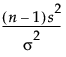Publication date: 03/23/2021

## Standard Deviation Test

Here is the formula for calculating the Test Statistic:The Test Statistic is distributed as a Chi-square variable with n - 1 degrees of freedom when the population is normal.

The Min PValue is the p-value of the two-tailed test, and is calculated as follows:

2*min(p1,p2)

where p1 is the lower one-tail p-value and p2 is the upper one-tail p-value.

Want more information? Have questions? Get answers in the JMP User Community (community.jmp.com).